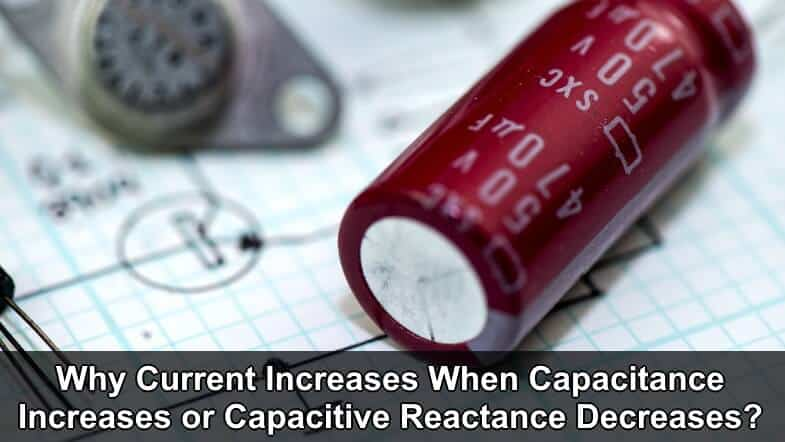# Why Current Increases When Capacitance Increases or Capacitive Reactance Decreases?

In Capacitive Circuit, Why the Circuit Current (I) Increases, When Capacitance (C) Increases or Inductive Reactance (XC) Decreases?

Next question from electrical and electronics engineering interviews question and answers series.

Explain the statement that ” In a capacitive circuit, when Capacitance (C) increases or capacitive reactance (XC) decreases, the Circuit Current (I) decreases“.Related Question: Why Current Decreases When Inductance or Inductive Reactance Increases?

Explanation:

We know that in DC circuits:

I = V / R,

But in case of AC circuits:

I = V / Z

Where “total resistance of AC circuits = Impedance = Z = √ (R2 + (XL – XC2)”

In case of Capacitive circuit:

• Z = √ (R2 + XC2)
• I = V / XC or I = V/Z

It shows that in a capacitive circuit, Current is inversely proportional to the capacitive reactance as well directly proportional to the capacitance “C” as capacitance and capacitive reactances “XC” are inversely proportional to each others.

Let’s check with an example to see how current reduced by capacitive reactance and increased by increasing the value of capacitor.

When Capacitance = 10 µF

Suppose a capacitive circuit where:

• Capacitance = C = 10µ Farads
• Voltage = V = 220 V
• Frequency f = 50 Hz

To find the capacitive reactance;

XC = 1 / 2πfC

XC = 1 / (2 x 3.1415 x 50 x 10×10-6)

XC = 318.3 Ω

Finally, current in capacitive circuit:

I = V / XC

I = 230V / 318.3 Ω

I = 0.72 A

Related Questions:

When Capacitance = 60 µF

Now we increased the value of capacitance (C) of a capacitor form 10 µF to 60 µF.

V = 220V, C = 60 µF, f = 50 Hz.

XC = 1 / 2πfC = 2 x 3.1415 x 50 x 60 µF = 53 Ω

I = V / XC = 230 V / 53 Ω

I = 4.34 A

Related Questions:

Conclusion:

We can see that, When capacitance (C) was 10µF, then circuit current were 0.72 A,

But when circuit capacitance increased from 10 µF to 60 µF, then the current increased from 0.72 A to 4.34 A.

Hence proved,

In a capacitive circuit, when capacitance increases, the capacitive reactance XC decreases which leads to increase the circuit current and vise versa.

In oral or verbal,

• Capacitive reactance is a kind of resistance. When resistance increases, the circuit current decreases and vice versa.
• Capacitance is inversely proportional to the capacitive reactance.

C 1 / XC

• Current is directly proportional to the capacitance and inversely proportional to capacitive reactance.

I C      and      I 1/XC